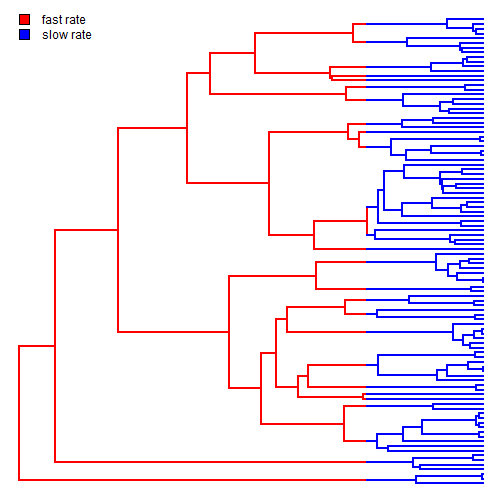## Thursday, September 24, 2015

### Modifications to brownieREML & new REML version of rateshift

I just updated the phytools function `brownieREML`, which implements a REML (restricted maximum likelihood) version of `brownie.lite` which is in turn based on Brian O'Meara et al. (2006; Evolution) method to test for multiple rates of continuous character evolution on the tree, where the branches associated with each rate regime are specified a priori by the user.

(1) Using the function `optimHess` I estimate the variance on the parameter estimates from the negative inverse of the Hessian matrix.

(2) I change some of the names of the elements of the list returned by `brownieREML` to match the corresponding elements from the function `brownie.lite`.

(3) Finally, I added an object class (`"brownieREML"`) and an S3 `print` method to the object returned by `brownieREML`.

Here's a very quick demo of these attributes:

``````library(devtools)
install_github("liamrevell/phytools",quiet=TRUE)
``````
``````library(phytools)
``````
``````## Loading required package: ape
``````
``````## set seed
set.seed(1)
## simulate multiple temporal regimes with different rates:
tree<-pbtree(n=100,scale=100)
mtree<-make.era.map(tree,c(0,75))
x<-sim.rates(mtree,setNames(c(10,1),1:2))
plot(mtree,ftype="off",colors=setNames(c("red","blue"),1:2))
c("fast rate","slow rate")),prompt=FALSE,x=0,y=Ntip(mtree))
````````````## fit using brownie.lite
fit.ml<-brownie.lite(mtree,x)
fit.ml
``````
``````## ML single-rate model:
##  s^2 se  a   k   logL
## value    2.7271  0.3856  8.5444  2   -323.1423
##
## ML multi-rate model:
##  s^2(1)  se(1)   s^2(2)  se(2)   a   k   logL
## value    14.2251 4.3892  0.7519  0.1221  8.5015  3   -285.7067
##
## P-value (based on X^2): 0
##
## R thinks it has found the ML solution.
``````
``````## fit using brownieREML
fit.reml<-brownieREML(mtree,x)
fit.reml
``````
``````## REML single-rate model:
##  s^2 se  k   logL
## value    2.7547  0.3915  1   -320.173
##
## REML multi-rate model:
##  s^2(1)  se(1)   s^2(2)  se(2)   k   logL
## value    14.9208 4.691   0.7511  0.1221  2   -281.947
##
## R thinks it has found the REML solution.
``````

The main reason for this update is to permit `brownieREML` to be used internally by `rateshift` for `rateshift(...,method="REML")`.

Here's more or less how that would look (along with the speed-up that results):

``````system.time(
fit.ml<-rateshift(tree,x,method="ML",nrates=2)
)
``````
``````## Optimization progress:
## |..........|
## Done.
``````
``````##    user  system elapsed
##  402.82    0.53  413.34
``````
``````fit.ml
``````
``````## ML 2-rate model:
##  s^2(1)  se(1)   s^2(2)  se(2)   k   logL
## value    13.7226 4.3593  0.7304  0.1196  4   -285.3171
##
## Shift point(s) between regimes (height above root):
##  1|2 se(1|2)
## value    75.6848 1.2073
##
## Model fit using ML.
##
## Frequency of best fit: 0.4
##
## R thinks it has found the ML solution.
``````
``````system.time(
fit.reml<-rateshift(tree,x,method="REML",nrates=2)
)
``````
``````## Optimization progress:
## |..........|
## Done.
``````
``````##    user  system elapsed
##   31.63    0.19   32.15
``````
``````fit.reml
``````
``````## ML 2-rate model:
##  s^2(1)  se(1)   s^2(2)  se(2)   k   logL
## value    14.4349 4.6601  0.7303  0.1196  4   -281.5724
##
## Shift point(s) between regimes (height above root):
##  1|2 se(1|2)
## value    75.6301 1.1231
##
## Model fit using REML.
##
## Frequency of best fit: 0.3
##
## R thinks it has found the REML solution.
``````

So, it is at least a little faster.